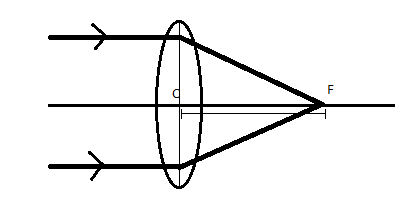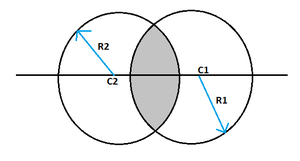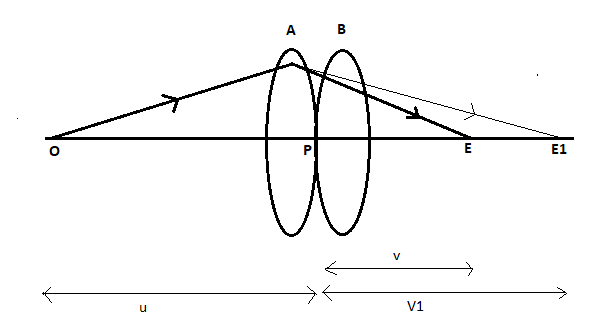# Power of a Lens

• Last Updated : 26 May, 2021

One of the most fascinating ideas of ray optics is the power of a lens. Simply stated, a lens’s capacity to bend light is its strength in Ray Optics. The ability of a lens to refract light that passes through it is proportional to its power. The converging ability of a convex lens is defined by its strength, while the diverging ability of a concave lens is defined by its diverging ability.

Are you aware of the connection between focal length and light ray bending? The number of light bends increases as the focal length decreases. As a result, the focal length of a lens is inversely proportional to its intensity. High optical strength is aided by a short focal range. Therefore, let’s first discuss some basic concepts related to the power of the lens.

### What is Focus and Focal Length of a Lens?

A lens is a piece of transparent material, mostly glass, that is used to focus or disperse a beam of light. It uses the refraction property of light. Refraction is the change observed in light’s path when it travels from one medium to another. The lens focuses the light to a point and forms the image. It’s mainly used as a contact lens in our specs, in the magnifying glass, microscopes, etc.

The focal length is a measure of how sharply the system converges or diverges light; it is the inverse of the lens’s optical power.

While a focus or focal point is a point at which light from infinity gets converged after passing through the lens. It is denoted by F.

Therefore, the distance or the length of the pole of the lens to its focal point is called its Focal length. It is denoted by f.

Based on the position of the focal point near a spherical lens, the focal length is of two types: Negative Focal length and Positive Focal length.

• Negative Focal Length: A negative focal length, means that the focal point is on the same side of the lens as the object. Generally, it is obtained in a concave lens as the parallel beam of light traveling parallel to the principal axis appears to diverge from the point or second focus, the focus is called virtual focus and its focal length is taken as negative.
• Positive Focal length: A positive focal length means that the focal point of the lens is on the other side of the lens from where the object is placed. Generally, it is observed in a convex lens as the parallel beam of light traveling parallel to the principal axis actually meets at a point, the focus is called real focus and its focal length is taken as positive.

Thus, a positive focal length denotes that the lens converges light, while a negative focal length denotes that the lens diverges light. A lens with a shorter focal length bends the rays more sharply, bringing them to a focus in a shorter distance or diverging them more quickly.### Power of a Lens

The ability of a lens to bend light is really what gives it its power in Ray Optics. The greater a lens’s strength, the greater its ability to refract light passing through it. Power defines the converging ability of a convex lens and the diverging ability of a concave lens. The number of light bends increases as the focal length reduces. As a result, we can assume that the strength of a lens is inversely proportional to its focal length. A short focal length, in essence, leads to high optical power.

Mathematically, the power of a lens is defined as:

Power (P) = 1 / Focal Length (f)

or

P = 1 / f

If the focal length is given in meters (m), the power of the lens is calculated in Diopters (D), as the lens’s unit of power is diopter. Another thing to remember is that the optical power of a converging lens is positive, while the optical power of a diverging lens is negative.

For instance, if the focal length of a lens is 15 cm, we get 0.15 m when we translate it to meters. Take the reciprocal of 0.15 to get the power of this prism, which is 6.67. As a result, the power of this lens is 6.67 D. This assumes that the power of a lens can be calculated using the radii of curvature of two surfaces and the refractive index of the lens material.

The power of a lens is inversely proportional to its focal length. Therefore, a short focal length lens has more power, whereas a lens of long focal length has less power.

• The power of a convex lens (converging lens) is positive as its focal length is positive.
• The power of a concave lens (diverging lens) is negative as its focal length is negative.
• The power of a plane glass plate is 0.

### Refractive index

A refractive index is a value that is often used in optical physics. Furthermore, there is no unit. The Refractive Index of a substance shows its ability to refract light. To better understand the definition of refractive index, one must first know the idea of refraction. Simply put, refraction occurs as light or other radiation changes the medium.

The ratio of the amplitude of an electromagnetic wave in a vacuum to its speed in another medium is referred to as the refractive index. It denotes the amount of refraction that will exist in a given substance. In addition, the refractive index in a vacuum is 1.

The normal can be described as the perpendicular line to the interface’s surface. In this case, a higher refractive index value indicates that the ray is bent towards the average. Refractive index refers to the calculation of the bending of a ray of light as it travels from one medium to another one. The majority of visible light refractive index values are between 1 and 2. Furthermore, refractive index values for infrared waves can be slightly higher.

Formula for Refractive Index:

The refractive index formula is given as follows:

n = c / v

where n is the refractive index of the medium, c is the speed of light when in a vacuum and v is the speed of light when in the medium.

### Power of a lens using the refractive index

There is a relation between the focal length of the lens and it’s the refractive index, it’s known as Lens maker’s formula. It includes the radius of curvatures of both surfaces. The lens is a part of the hollow sphere of glass, Radius of curvature of the lens is the radius of that sphere. Each lens has two radii of curvature.Radii of curvature of a convex lens

According to the Lens maker’s formula:

1/f = (n-1) × (1/R1 – 1/R2)

where, n is the Refractive index of the material, f is the focal length of the lens, R1 is the radius of curvatures of first surface and R2 is the Radius of curvature of second surface.

Also the power of a lens is given by,

P = 1 / f

Now, from both the above equations:

P = (n-1) × (1/R1 – 1/R2)

This is the required relation between the power and the refractive index of the lens. This formula can be used to find the power of the lens using the refractive index of the material and radius of curvatures of the lens.

## Power of combination of lenses

Two or more lenses can be combined in order to increase or decrease the power of lenses. The formula of the combination is simple and is explained with the help of an image.Combination of lenses

Let there be two lenses A and B with focal lengths f1 and f2 respectively. These two lenses are placed in contact with each other such that their principal axes coincide with each other. An object is placed at O on the principal axis of the combination. Lens A produces an image of the object at E1. This image acts as an object for lens B and the final image is formed at E.

PO = u, i.e. object distance for lens A

PE = v, i.e. final image distance

PE1 = V1, i.e. image distance for lens A and object distance for lens B.

Using Lens formula on the image formed by lens A:

1/v1 – 1/u = 1/f1                                                                                                                                                                                            ……(1)

Using Lens formula on the image formed by lens B:

1/v – 1/v1 = 1/f2                                                                                                                                                                                            ……(2)

Adding equation (1) and (2) as:

1/v – 1/u = 1/f1 + 1/f2                                                                                                                                                                                  ……(3)

Replace the combination with a single lens of focal length F, such that the final image is formed at E.

1/v – 1/u = 1/F                                                                                                                                                                                               ……(4)

Now from equation (1) and (2) as:

1/F = 1/f1 + 1/f2                                                                                                                                                                                            ……(5)

where, F is the focal length of the combination of lenses A and B.

Since,

P = 1 / f

Therefore, equation (5) changes to

P = P1 + P2

where, P is the power of the combination of lenses, P1 is the Power of lens A, P2 is the Power of lens B.

This is the formula for the combined power of lenses. Proper sign convention needs to be followed when substituting the values of P1 and P2.

### Sample Problems

Problem 1: How does the power of a lens change if its focal length is doubled?

Solution:

Power gets halved as Power is inversely proportional to focal length.

Problem 2: What is the power of convex lens (with sign) of focal length 40cm?

Solution:

Since, Power = 1 / f

Substituting the given values as,

P = 100/40

= 2.5D

Since it’s a convex lens, so power will be positive.

Thus, the power of convex lens is +2.5D.

Problem 3: Identify the type of lens, and its focal length if its power is 0.2D.

Solution:

Since the focal length, f = 1 / Power (P)

Therefore, substituting the given values in the above expression as:

f = 1 / (0.2D)

= 5 m

Since the power is positive, therefore given lens is a convex lens.

Problem 4: Sunglasses have curved surface but still do not have any power. Why?

Solution:

Sunglasses have two curved surfaces, one is convex and another is concave. Both surfaces are of equal power but of opposite signs, so both the power cancels out each other and resultant power is 0.

Problem 5: A convex lens of focal length 50 cm is in contact with a concave lens of 20 cm focal length. Find the power of the combination of lenses.

Solution:

For a combination of lenses,

P = P1 + P2

or

P = 1/f1 + 1/f2

P = 100/50 + 100/(-20)                                                                                                 (concave lenses have negative focal length)

= -3 D

My Personal Notes arrow_drop_up